# RD Sharma Class 8 Solutions Chapter 1 Rational Numbers Ex 1.3

In this chapter, we provide RD Sharma Class 8 Solutions Chapter 1 Rational Numbers Ex 1.3 for English medium students, Which will very helpful for every student in their exams. Students can download the latest RD Sharma Class 8 Solutions Chapter 1 Rational Numbers Ex 1.3 Maths pdf, free RD Sharma Class 8 Solutions Chapter 1 Rational Numbers Ex 1.3 book pdf download. Now you will get step by step solution to each question.

### RD Sharma Solutions for Class 8 Chapter 1 Rational Numbers Ex 1.3 Download PDF

Question 1.
Subtract the first rational number from the second in each of the following :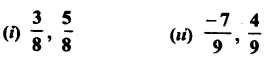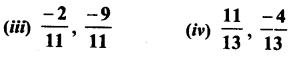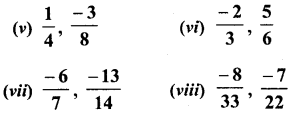Solution: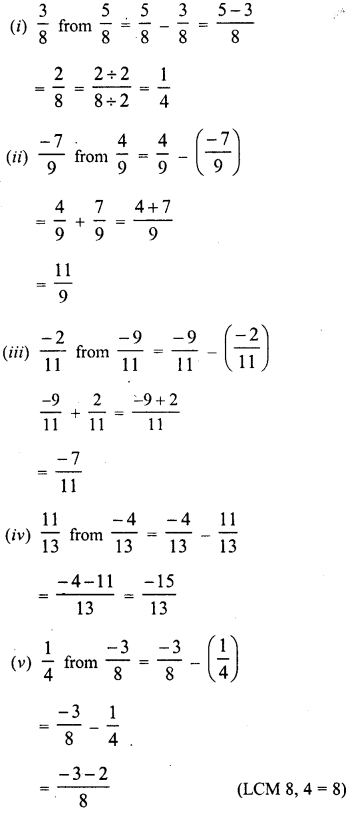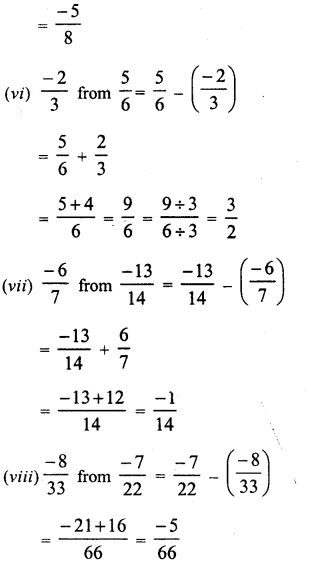Question 2.
Evaluate each of the following :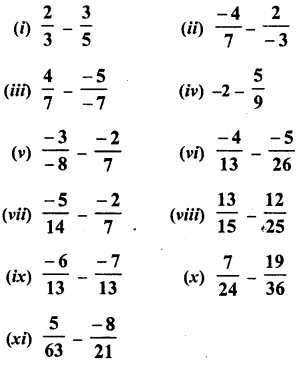Solution: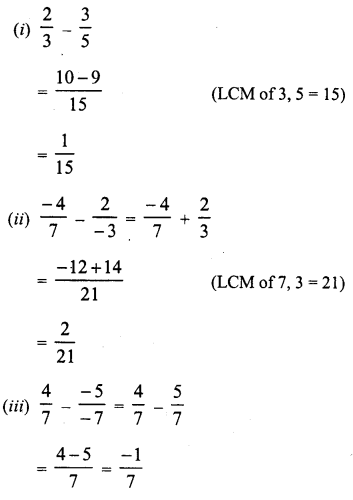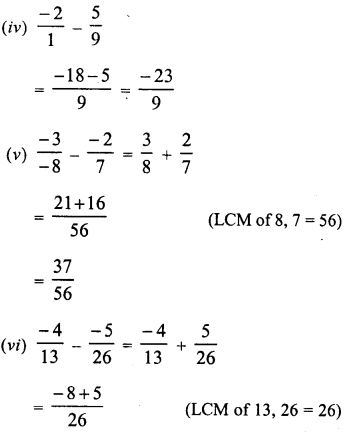Question 3.
The sum of two numbers is 59. If one of the numbers is 13, find the other.
Solution:Question 4.
The sum of two numbers is −13. If one of the numbers is −123, find the other.
Solution:Question 5.
The sum of two numbers is −43. If one of the number is -5, find the
Solution:Question 6.
The sum of two rational numbers is -8. If one of the numbers is −157 find the other.
Solution:Question 7.
What should be added to so as to −78 get 59 ?
Solution:Question 8.
What number should be added to −511 so as to get 263 ?
Solution:Question 9.
What number should be added to −57 to get −23 ?
Solution:Question 10.
What number should be subtracted from −53 to get 56 ?
Solution:Question 11.
What number should be subtracted from 37 to get 54 ?
Solution:Question 12.
What should be added to (23+35) to get −1215 ?
Solution: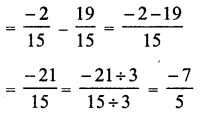Question 13.
What should be added to (12+13+15) to get 3 ?
Solution: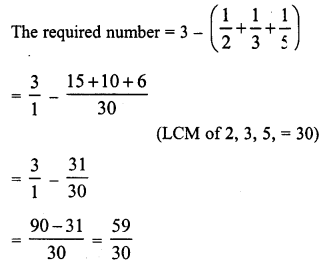Question 14.
What should be subtracted from (34−23) to get −16 ?
Solution:Question 15.
Fill in the blanks :Solution: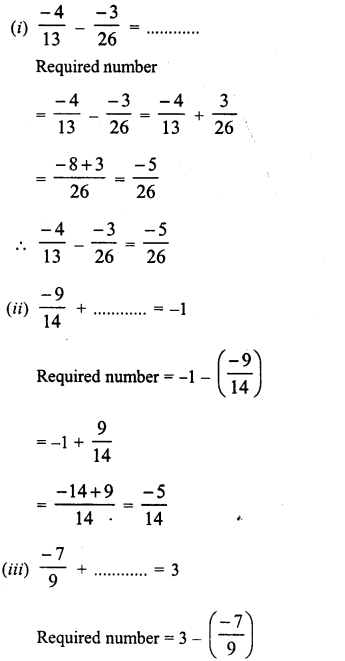All Chapter RD Sharma Solutions For Class 8 Maths

—————————————————————————–

All Subject NCERT Exemplar Problems Solutions For Class 8

All Subject NCERT Solutions For Class 8

*************************************************

I think you got complete solutions for this chapter. If You have any queries regarding this chapter, please comment on the below section our subject teacher will answer you. We tried our best to give complete solutions so you got good marks in your exam.

If these solutions have helped you, you can also share rdsharmasolutions.in to your friends.